|

# Gorenje集团与赫曼德·家赋新 共生型联盟

6月25日，家电行业的传统格局正在被一场巨头间的联手所颠覆。事件的主角是国际奢品家电巨头Gorenje集团和欧洲进口大家居领域巨头Hermand Haus赫曼德·家。当天正值赫曼德·家十五周年庆典，在盛典上，两巨头共同宣布：将联手打造面向未来的“共生型联盟”，在战略上从“单赢逻辑”转向“共赢逻辑”，在产品迭代、消费场景、用户体验等方面共同为消费者创造“高品质的艺术生活”。“无论是Gorenje还是Hermand Haus赫曼德·家，他们都把自己的边界打开，互相赋能，互为主体，他们将是全新价值的塑造者，对于家电行业来说，这种‘共生型联盟’打破了传统生态格局，对整个行业的发展有着里程碑意义。”行业专家如是分析。

共创、共享、共赢，两巨头联盟赋新“共生型组织”

在当天盛典上，Gorenje集团与Hermand Haus赫曼德·家共同签署战略合作协议，组成“进口高端家居领域联盟”。Gorenje集团表示：此次两大集团展开战略合作，将共同开创、合力打造共生共赢生态系统，通过最优“组合”，实现从整厨设计、家电嵌入、到安装交付的“无忧一体化”系统，为消费者打造全方位完美厨房空间。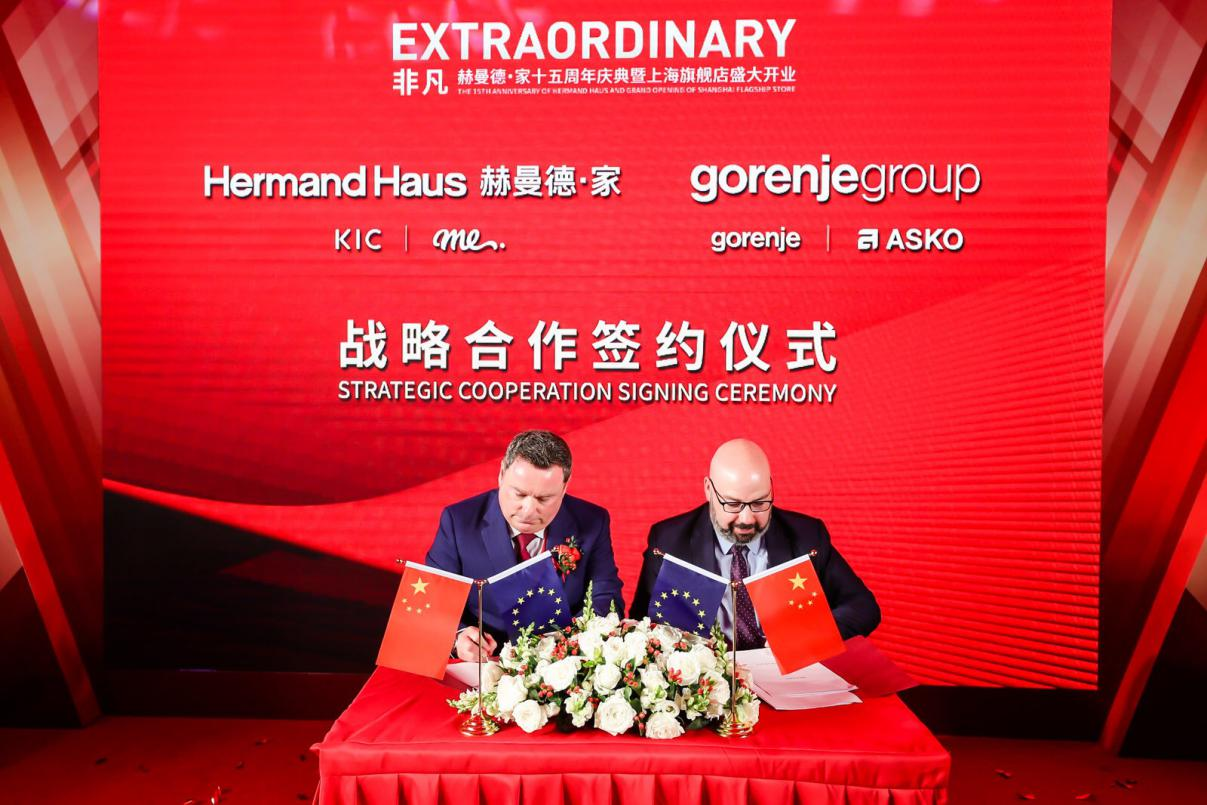【Gorenje集团与Hermand Haus赫曼德·家签约现场，赋新“共生型联盟”】

“此次合作，是一次全新的探索，Hermand Haus持续发现、研究并引进多元化的、与时俱进的、市场所需的、引领潮流的生活方式，服务生活，致敬生活，让极致触手可及。”Hermand Haus赫曼德·家相关领导表示。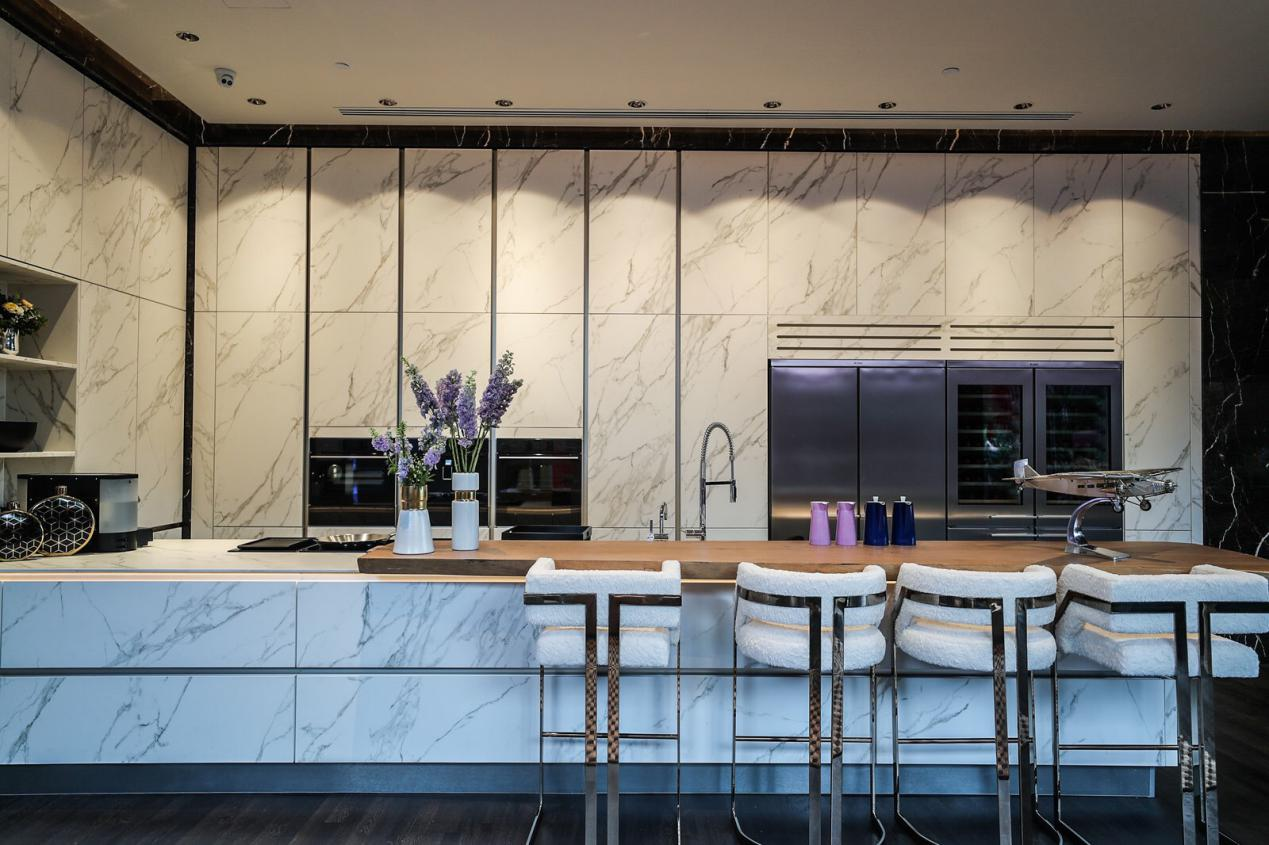【Hermand Haus赫曼德·家旗下品牌KIC欧洲整体厨房·衣柜衣帽间将采用Gorenje集团旗下品牌ASKO产品】

在“厨房经济”与“消费升级”的双重大势下，品牌只有维持在一种具有张力的开放性系统中，才能不断与外界进行信息、资源、能量等的互换，进而实现自身的生存与发展。作为“共创共享”全新模式的倡导者，Gorenje集团与Hermand Haus赫曼德·家对整个行业进行了一场颠覆。

“异业共享是经济发展的必然。通过共享融合、相互渗透，品牌可以获得更多的资源、从而赢得更广阔的市场。”Gorenje集团(中国)总经理Leon表示，“好的合作伙伴与模式，将让双方进入一场良性循环。”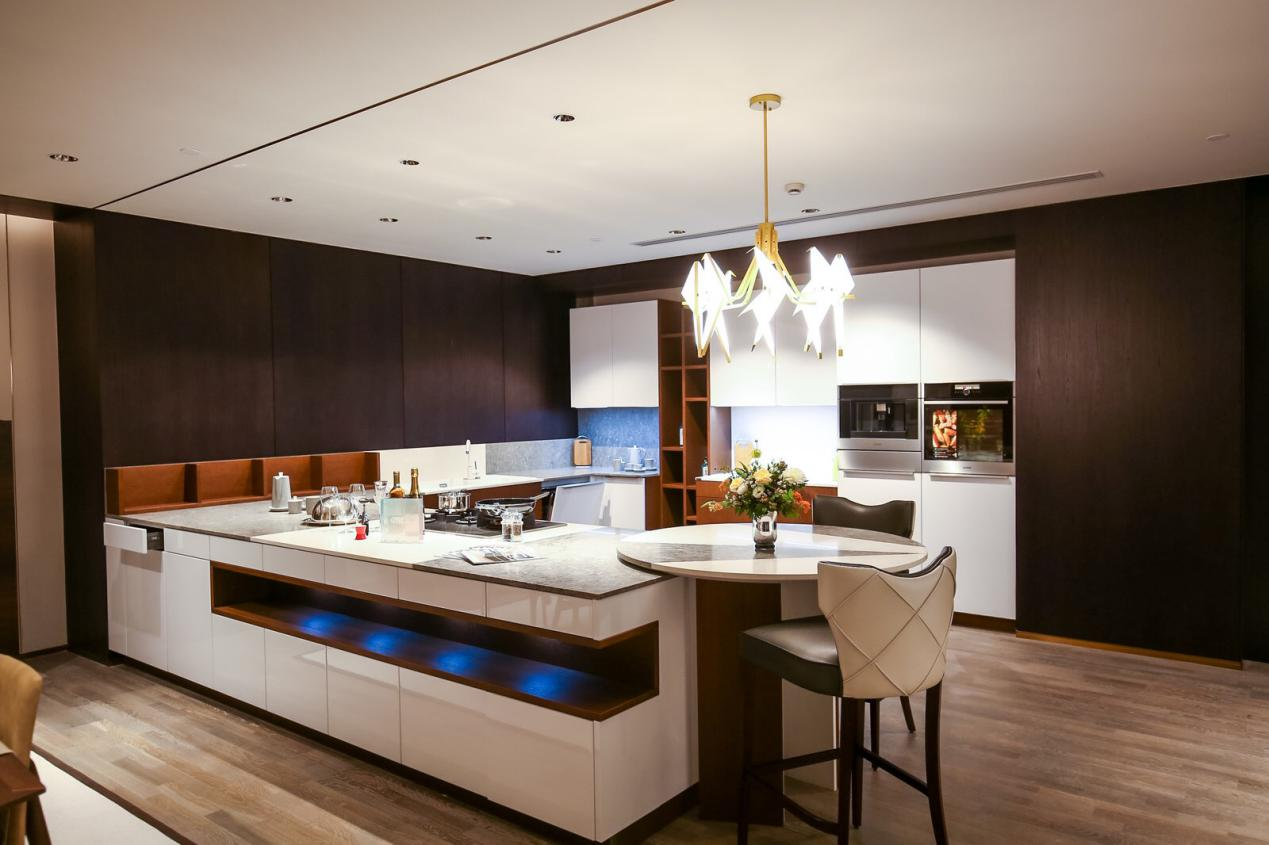【Hermand Haus赫曼德·家旗下欧洲进口全屋定制品牌me将采用Gorenje集团旗下品牌gorenje产品】

据了解，通过此次战略合作，双方在产业链上将进行更为深入的共享、融合。对于消费者而言，实现了从橱柜到厨电的“一站式”：一站式购买、一站式设计、一站式服务。不仅省去以往橱柜与厨电搭配不协调的烦恼，提升整体厨房的设计感、舒适度；更可在后续保养中，直接对接两大品牌，这将更有保障。

互相赋能，定义奢品家电

“从品牌高度到产品契合度，Gorenje集团与Hermand Haus赫曼德·家都相得益彰。通过此次战略合作，我们在‘内’，将以科技重新定义体验式场景，用细节成就奢华；在‘外’，践行简约而不简单的设计理念，共同打造舒心的厨房空间，共建温馨家。”Gorenje集团(中国)总经理Leon告诉记者。

Gorenje集团是历史最悠久的现代家电集团之一。目前拥有gorenje（戈兰尼）、ASKO（雅士高）2个全球品牌和6个区域品牌，产品销售90多个国家和地区，gorenje（戈兰尼）在斯洛文尼亚语中，是“燃烧”的意思，不管是对食物，还是对生活，都有着火一般的热情。gorenje（戈兰尼）设计感极强，与世界级的知名设计师ORA- ÏTO 、Philippe Starck 等推出过一系列令人瞩目的奢品家电潮品。譬如，德国销量第一的彩色复古冰箱系列等。在欧洲，gorenje更被誉为集欧洲科技和欧洲设计之大成，多款设计获得红点奖。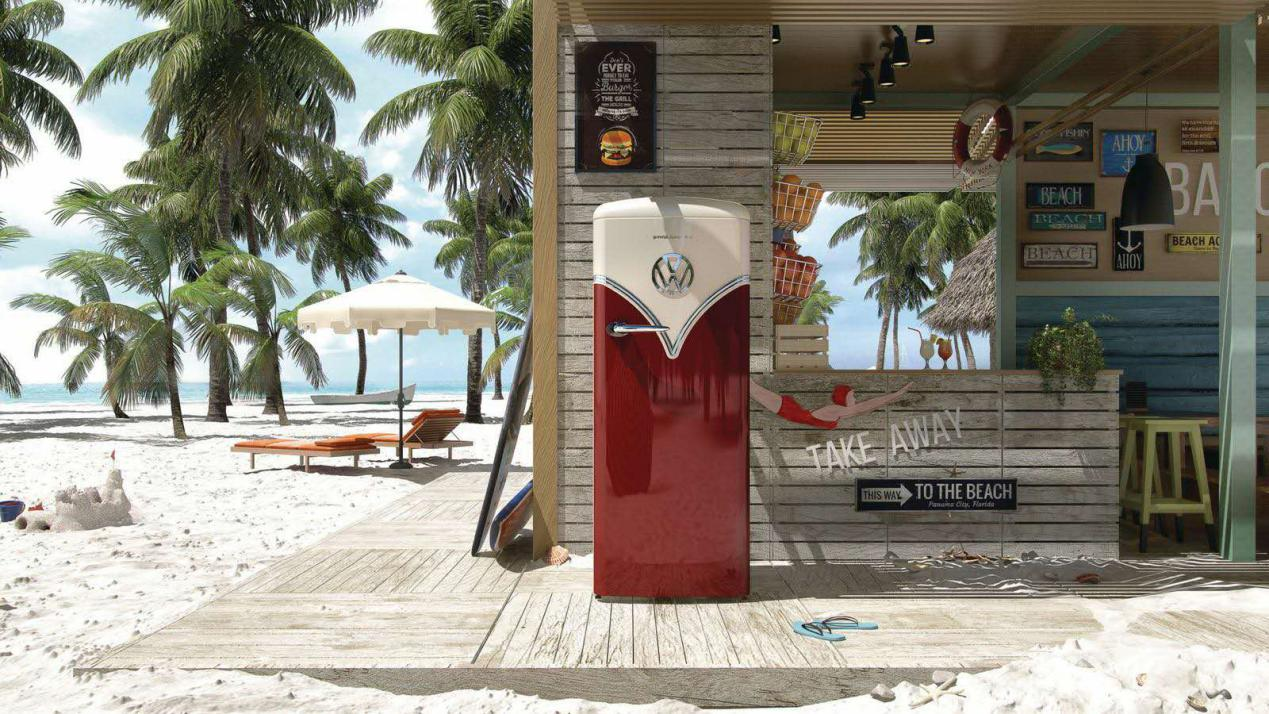【gorenje复古冰箱大众联名款】

另一“当家花旦”ASKO（雅士高）则是北欧顶级奢侈品家电品牌，已经走过百年历史。不同于行业设计大多偏爱的复杂繁琐风格，ASKO（雅士高）产品的设计一直凸显北欧极简风格，也是第一个将“斯堪的纳维亚”式设计引入到家电设计的品牌。而在材质配置和科技上，更是保持着一流水准，力求家用产品也能达到至少20年的使用寿命。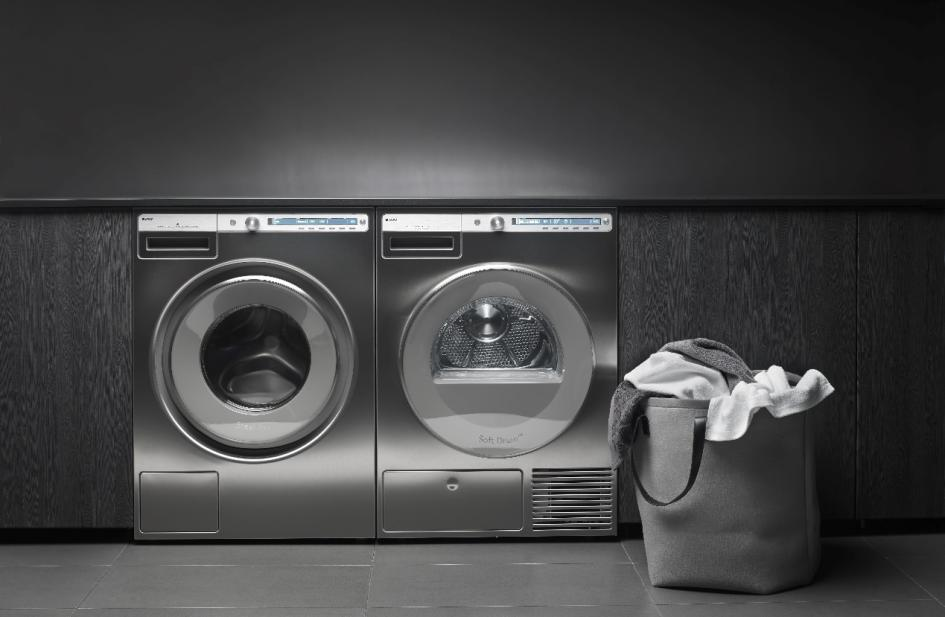【ASKO逻辑系列洗衣机干衣机】

同样，作为合作伙伴的Hermand Haus赫曼德·家也是“大有来头”。成立于2005年的Hermand Haus赫曼德·家，是一家具有15年行业经验积累，拥有自主品牌的欧洲原装进口家居产品集成服务商，一直秉承“Made in Europe，Loved in China”的品牌核心理念，致力于为中国更多追求高品质生活的精英消费者提供欧洲优质的全品类进口定制家居， Hermand Haus赫曼德·家采用欧洲一流的生产技术，并结合时下流行家居潮流，推出符合当下高端消费群体需求的产品，满足中国塔尖圈层对生活品质的极致追求。

对于中国高端家居行业来说，这样的强强组合目前还是空白。“与谁共创，如何共生，怎样共赢，这是我们一直思考的问题，在这样的时空，我们不约而同选择了对方。除了价值观高度一致外，对未来的共同期许，也是我们合作的强大动力，我们相信以欧洲的品质、标准、精湛工艺以及独到的艺术灵感，一定能为中国精英家庭打造独具质感、温馨幸福的一站式居家体验。”Gorenje集团(中国)总经理Leon对记者表示。

`声明：本文由入驻焦点开放平台的作者撰写，除焦点官方账号外，观点仅代表作者本人，不代表焦点立场错误信息举报电话： 400-099-0099，邮箱：jubao@vip.sohu.com，或点此进行意见反馈，或点此进行举报投诉。`A B C D E F G H J K L M N P Q R S T W X Y Z
A - B - C - D - E
• A
• 鞍山
• 安庆
• 安阳
• 安顺
• 安康
• 澳门
• B
• 北京
• 保定
• 包头
• 巴彦淖尔
• 本溪
• 蚌埠
• 亳州
• 滨州
• 北海
• 百色
• 巴中
• 毕节
• 保山
• 宝鸡
• 白银
• 巴州
• C
• 承德
• 沧州
• 长治
• 赤峰
• 朝阳
• 长春
• 常州
• 滁州
• 池州
• 长沙
• 常德
• 郴州
• 潮州
• 崇左
• 重庆
• 成都
• 楚雄
• 昌都
• 慈溪
• 常熟
• D
• 大同
• 大连
• 丹东
• 大庆
• 东营
• 德州
• 东莞
• 德阳
• 达州
• 大理
• 德宏
• 定西
• 儋州
• 东平
• E
• 鄂尔多斯
• 鄂州
• 恩施
F - G - H - I - J
• F
• 抚顺
• 阜新
• 阜阳
• 福州
• 抚州
• 佛山
• 防城港
• G
• 赣州
• 广州
• 桂林
• 贵港
• 广元
• 广安
• 贵阳
• 固原
• H
• 邯郸
• 衡水
• 呼和浩特
• 呼伦贝尔
• 葫芦岛
• 哈尔滨
• 黑河
• 淮安
• 杭州
• 湖州
• 合肥
• 淮南
• 淮北
• 黄山
• 菏泽
• 鹤壁
• 黄石
• 黄冈
• 衡阳
• 怀化
• 惠州
• 河源
• 贺州
• 河池
• 海口
• 红河
• 汉中
• 海东
• 怀来
• I
• J
• 晋中
• 锦州
• 吉林
• 鸡西
• 佳木斯
• 嘉兴
• 金华
• 景德镇
• 九江
• 吉安
• 济南
• 济宁
• 焦作
• 荆门
• 荆州
• 江门
• 揭阳
• 金昌
• 酒泉
• 嘉峪关
K - L - M - N - P
• K
• 开封
• 昆明
• 昆山
• L
• 廊坊
• 临汾
• 辽阳
• 连云港
• 丽水
• 六安
• 龙岩
• 莱芜
• 临沂
• 聊城
• 洛阳
• 漯河
• 娄底
• 柳州
• 来宾
• 泸州
• 乐山
• 六盘水
• 丽江
• 临沧
• 拉萨
• 林芝
• 兰州
• 陇南
• M
• 牡丹江
• 马鞍山
• 茂名
• 梅州
• 绵阳
• 眉山
• N
• 南京
• 南通
• 宁波
• 南平
• 宁德
• 南昌
• 南阳
• 南宁
• 内江
• 南充
• P
• 盘锦
• 莆田
• 平顶山
• 濮阳
• 攀枝花
• 普洱
• 平凉
Q - R - S - T - W
• Q
• 秦皇岛
• 齐齐哈尔
• 衢州
• 泉州
• 青岛
• 清远
• 钦州
• 黔南
• 曲靖
• 庆阳
• R
• 日照
• 日喀则
• S
• 石家庄
• 沈阳
• 双鸭山
• 绥化
• 上海
• 苏州
• 宿迁
• 绍兴
• 宿州
• 三明
• 上饶
• 三门峡
• 商丘
• 十堰
• 随州
• 邵阳
• 韶关
• 深圳
• 汕头
• 汕尾
• 三亚
• 三沙
• 遂宁
• 山南
• 商洛
• 石嘴山
• T
• 天津
• 唐山
• 太原
• 通辽
• 铁岭
• 泰州
• 台州
• 铜陵
• 泰安
• 铜仁
• 铜川
• 天水
• 天门
• W
• 乌海
• 乌兰察布
• 无锡
• 温州
• 芜湖
• 潍坊
• 威海
• 武汉
• 梧州
• 渭南
• 武威
• 吴忠
• 乌鲁木齐
X - Y - Z
• X
• 邢台
• 徐州
• 宣城
• 厦门
• 新乡
• 许昌
• 信阳
• 襄阳
• 孝感
• 咸宁
• 湘潭
• 湘西
• 西双版纳
• 西安
• 咸阳
• 西宁
• 仙桃
• 西昌
• Y
• 运城
• 营口
• 盐城
• 扬州
• 鹰潭
• 宜春
• 烟台
• 宜昌
• 岳阳
• 益阳
• 永州
• 阳江
• 云浮
• 玉林
• 宜宾
• 雅安
• 玉溪
• 延安
• 榆林
• 银川
• Z
• 张家口
• 镇江
• 舟山
• 漳州
• 淄博
• 枣庄
• 郑州
• 周口
• 驻马店
• 株洲
• 张家界
• 珠海
• 湛江
• 肇庆
• 中山
• 自贡
• 资阳
• 遵义
• 昭通
• 张掖
• 中卫

1室1厅1厨1卫1阳台

1
2
3
4
5

0
1
2

1

1

0
1
2
3报名成功，资料已提交审核A B C D E F G H J K L M N P Q R S T W X Y Z
A - B - C - D - E
• A
• 鞍山
• 安庆
• 安阳
• 安顺
• 安康
• 澳门
• B
• 北京
• 保定
• 包头
• 巴彦淖尔
• 本溪
• 蚌埠
• 亳州
• 滨州
• 北海
• 百色
• 巴中
• 毕节
• 保山
• 宝鸡
• 白银
• 巴州
• C
• 承德
• 沧州
• 长治
• 赤峰
• 朝阳
• 长春
• 常州
• 滁州
• 池州
• 长沙
• 常德
• 郴州
• 潮州
• 崇左
• 重庆
• 成都
• 楚雄
• 昌都
• 慈溪
• 常熟
• D
• 大同
• 大连
• 丹东
• 大庆
• 东营
• 德州
• 东莞
• 德阳
• 达州
• 大理
• 德宏
• 定西
• 儋州
• 东平
• E
• 鄂尔多斯
• 鄂州
• 恩施
F - G - H - I - J
• F
• 抚顺
• 阜新
• 阜阳
• 福州
• 抚州
• 佛山
• 防城港
• G
• 赣州
• 广州
• 桂林
• 贵港
• 广元
• 广安
• 贵阳
• 固原
• H
• 邯郸
• 衡水
• 呼和浩特
• 呼伦贝尔
• 葫芦岛
• 哈尔滨
• 黑河
• 淮安
• 杭州
• 湖州
• 合肥
• 淮南
• 淮北
• 黄山
• 菏泽
• 鹤壁
• 黄石
• 黄冈
• 衡阳
• 怀化
• 惠州
• 河源
• 贺州
• 河池
• 海口
• 红河
• 汉中
• 海东
• 怀来
• I
• J
• 晋中
• 锦州
• 吉林
• 鸡西
• 佳木斯
• 嘉兴
• 金华
• 景德镇
• 九江
• 吉安
• 济南
• 济宁
• 焦作
• 荆门
• 荆州
• 江门
• 揭阳
• 金昌
• 酒泉
• 嘉峪关
K - L - M - N - P
• K
• 开封
• 昆明
• 昆山
• L
• 廊坊
• 临汾
• 辽阳
• 连云港
• 丽水
• 六安
• 龙岩
• 莱芜
• 临沂
• 聊城
• 洛阳
• 漯河
• 娄底
• 柳州
• 来宾
• 泸州
• 乐山
• 六盘水
• 丽江
• 临沧
• 拉萨
• 林芝
• 兰州
• 陇南
• M
• 牡丹江
• 马鞍山
• 茂名
• 梅州
• 绵阳
• 眉山
• N
• 南京
• 南通
• 宁波
• 南平
• 宁德
• 南昌
• 南阳
• 南宁
• 内江
• 南充
• P
• 盘锦
• 莆田
• 平顶山
• 濮阳
• 攀枝花
• 普洱
• 平凉
Q - R - S - T - W
• Q
• 秦皇岛
• 齐齐哈尔
• 衢州
• 泉州
• 青岛
• 清远
• 钦州
• 黔南
• 曲靖
• 庆阳
• R
• 日照
• 日喀则
• S
• 石家庄
• 沈阳
• 双鸭山
• 绥化
• 上海
• 苏州
• 宿迁
• 绍兴
• 宿州
• 三明
• 上饶
• 三门峡
• 商丘
• 十堰
• 随州
• 邵阳
• 韶关
• 深圳
• 汕头
• 汕尾
• 三亚
• 三沙
• 遂宁
• 山南
• 商洛
• 石嘴山
• T
• 天津
• 唐山
• 太原
• 通辽
• 铁岭
• 泰州
• 台州
• 铜陵
• 泰安
• 铜仁
• 铜川
• 天水
• 天门
• W
• 乌海
• 乌兰察布
• 无锡
• 温州
• 芜湖
• 潍坊
• 威海
• 武汉
• 梧州
• 渭南
• 武威
• 吴忠
• 乌鲁木齐
X - Y - Z
• X
• 邢台
• 徐州
• 宣城
• 厦门
• 新乡
• 许昌
• 信阳
• 襄阳
• 孝感
• 咸宁
• 湘潭
• 湘西
• 西双版纳
• 西安
• 咸阳
• 西宁
• 仙桃
• 西昌
• Y
• 运城
• 营口
• 盐城
• 扬州
• 鹰潭
• 宜春
• 烟台
• 宜昌
• 岳阳
• 益阳
• 永州
• 阳江
• 云浮
• 玉林
• 宜宾
• 雅安
• 玉溪
• 延安
• 榆林
• 银川
• Z
• 张家口
• 镇江
• 舟山
• 漳州
• 淄博
• 枣庄
• 郑州
• 周口
• 驻马店
• 株洲
• 张家界
• 珠海
• 湛江
• 肇庆
• 中山
• 自贡
• 资阳
• 遵义
• 昭通
• 张掖
• 中卫• 手机• 分享
• 设计
免费设计
• 计算器
装修计算器
• 入驻
合作入驻
• 联系
联系我们
• 置顶
返回顶部